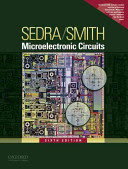### Electronics 1

#### Course Description

Introduction. Basic characteristics of analog and digital circuits. Electrical characteristics of semiconductors, charge carriers, current conduction in semiconductors. pn and Schottky diode. Basic diode circuits in analog electronics (rectifiers, regulators, limiting circuits). MOS structure and MOSFET. Various FET types. Basic FET amplifier circuits. Basic CMOS logic circuits (inverter, NAND and NOR circuits, bistable multivibrator). Bipolar junction transistor (BJT). Basic BJT amplifier circuits. Differential amplifier. BJT switch. ECL logic circuits. Analysis of electronic circuits by CAD tools.

#### Learning Outcomes

1. explain the structure and operation principle of basic semiconductor devices
2. explain the electrical charcteristics of basic semiconductor devices
3. analyze basic electronic amplifiers
4. compare the amplifiers with different electronic devices
5. analyze CMOS logic circuits
6. analyze of electronic circuits using electronic computers
7. apply basic measurements in electronic laboratory

#### Forms of Teaching

Lectures

Lectures

Exercises

Problem solving

Laboratory

Lab excercises

Continuous Assessment Exam
Laboratory Exercises 2 % 12 % 2 % 12 %
Mid Term Exam: Written 0 % 44 % 0 %
Final Exam: Written 8 % 44 %
Exam: Written 20 % 50 %
Exam: Oral 38 %
##### Comment:

Before final exam students must have completed all laboratory exercises.

#### Week by Week Schedule

1. Application and importance of electronics, Introduction to amplifiers (gain, input and output resistance)
2. Introduction to amplifiers (gain, input and output resistance), Introduction to digital electronics
3. Intrinsic and extrinsic semiconductors, Carrier concentrations, electrons and holes
4. Carrier concentrations, electrons and holes, Current flow in semiconductors (drift and diffusion current)
5. Operating principle, Electrical characteristics
6. Small-signal model, Optoelectronic electron devices, Basic rectifier circuits
7. Structure and operating principle of MOSFET and JFET, Electrical characteristics, Small-signal model, Definition and stabilization of bias operating point
8. Midterm exam
9. Basic amplifier configurations: common source, common gate, common drain, Calculation of gain, input and output resistances of basic amplifier configurations, Calculation of output voltage swing
10. CMOS inverter, Combinational CMOS circuits, Sequential CMOS circuits
11. Structure and operating principle of BJT, Electrical characteristics, Small-signal model
12. Definition and stabilization of bias operating point, Basic amplifier configurations: common emitter, common base, common collector, Calculation of gain, input and output resistances of basic amplifier configurations, Calculation of output voltage swing
13. Basic amplifier configurations: common emitter, common base, common collector, Calculation of gain, input and output resistances of basic amplifier configurations
14. Inverters with bipolar transistors, Current switch with BJT and basic ECL circuit
15. Final exam

#### Study Programmes

[FER3-EN] Electrical Engineering and Information Technology - study
(3. semester)

#### Literature

Ž. Butković, J. Divković-Pukšec, A. Barić (2018.), Elektronika 1, Fakutet elektrotehnike i računarstva, Zagreb - interna skripta, Fakutet elektrotehnike i računarstva Sveučilišta u ZagrebuA.S. Sedra, K.C. Smith (2011.), Microelectronic Circuits, 6th ed., Oxford University Press
R.C. Jaeger, T.N. Blalock (2011.), Microelectronic Circuit Design, 4th ed., McGraw-Hill

#### General

ID 209725
Winter semester
6 ECTS
L1 English Level
L1 e-Learning
60 Lectures
0 Seminar
15 Exercises
12 Laboratory exercises
0 Project laboratory